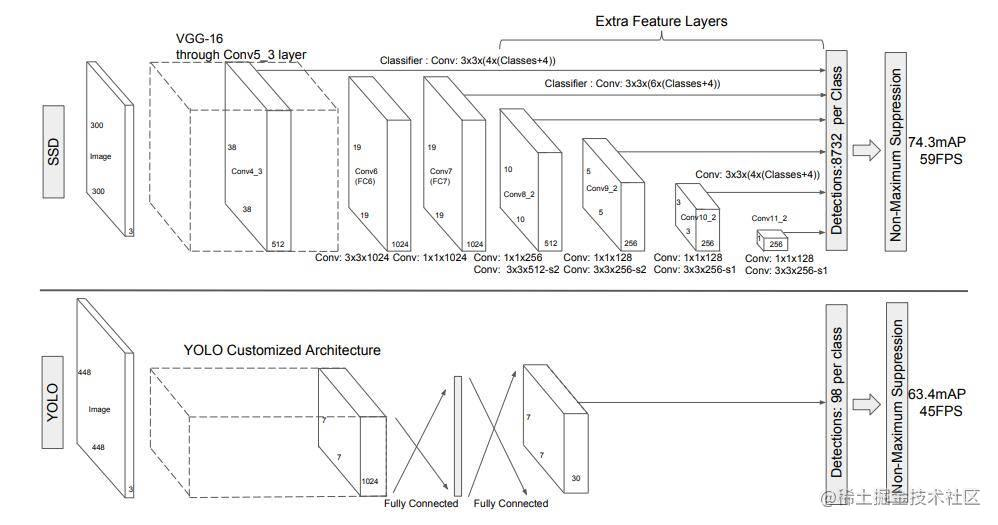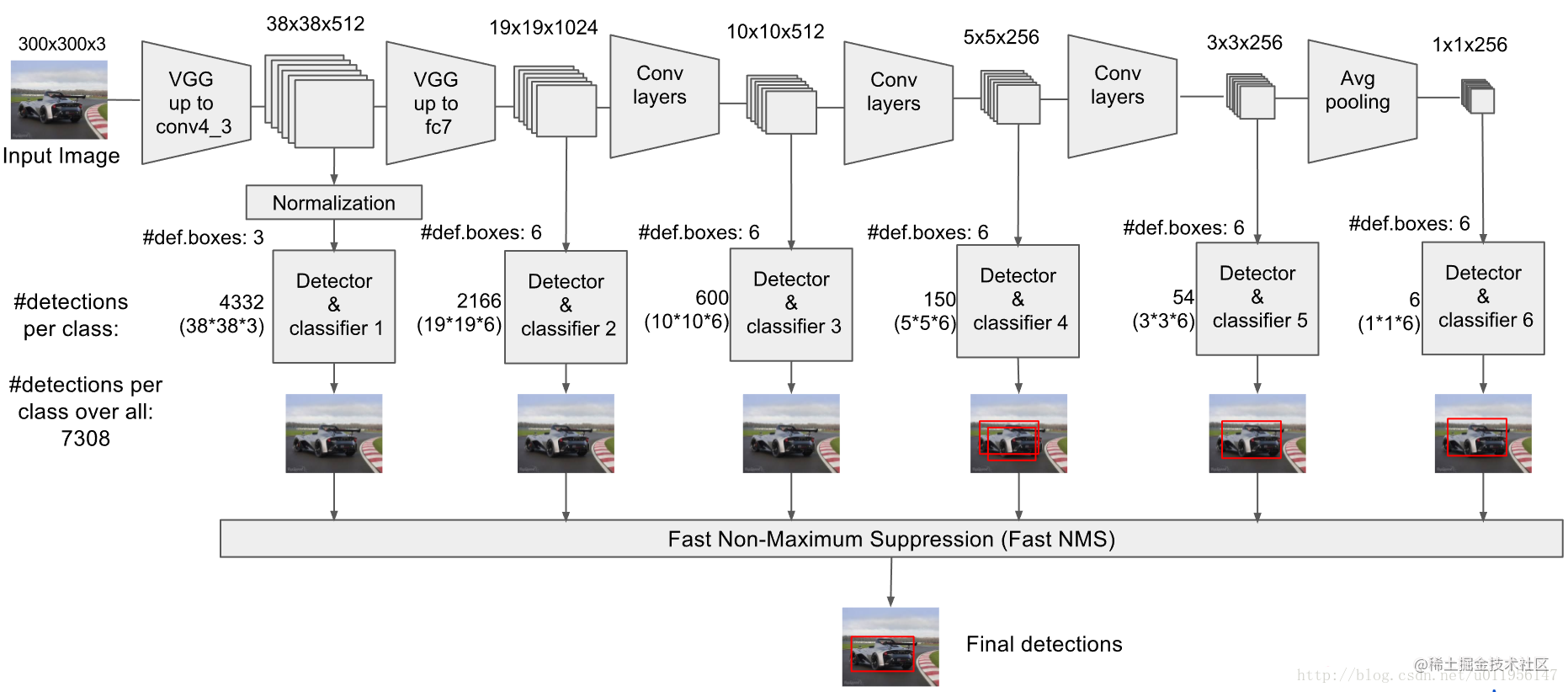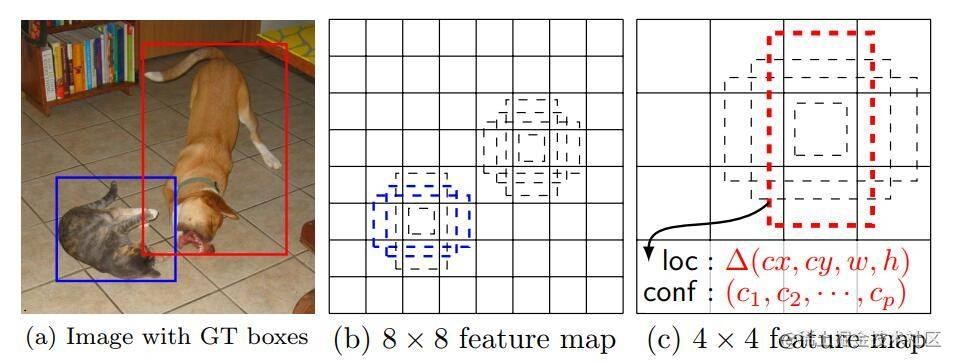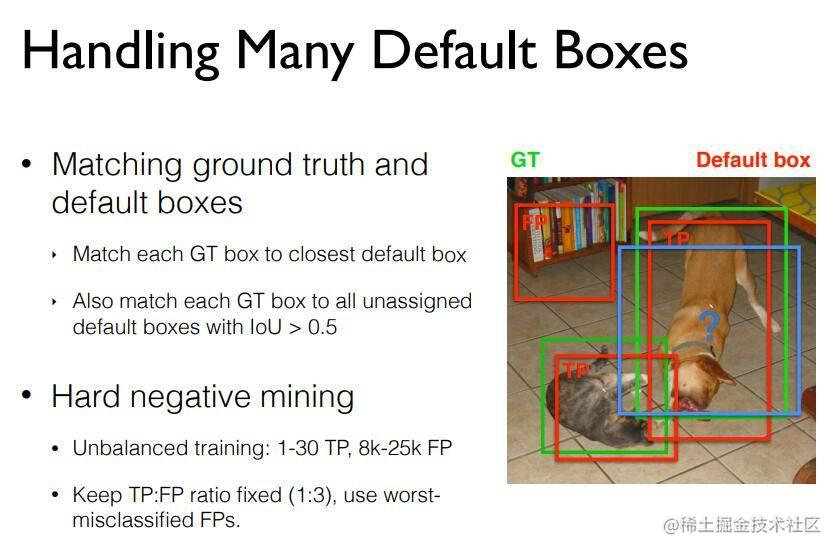# 网络构架

SSD的原始网络构架建议还是以论文为准，毕竟平时我们接触到的都是各种魔改版(也就是所谓的换了backbone，例如最常见的SSD-mobilenetv2)，虽然与原版大同小异，不过对于理解来说，会增大我们理解的难度，因此，完全有必要看一遍原始的论文描述。

SSD在论文中是采取的VGG网络作为主干结构，但是去除了VGG中的最后几层(也就是我们经常说的分类层)，随后添加了一些新的内容(在原文中叫做auxiliary structure)，这些层分别是：

• 额外的特征提取层(Extra Feature Layers)，作用就是和原本backbone的层相结合共同提取出不同尺寸的特征信息，相当于加强了之前的backbone，使其网络更深，提取能力更加强大。
• 坐标位置回归层(regression hearders)，结构与分类层相仿，只是输出的通道略有不同，通过对不同尺度的特征图进行卷积，输出的是每个特征图中每个坐标对应的default boxes的偏移坐标(文章中称为shape offset)。## 用于检测的多尺度特征图## default box

We associate a set of default bounding boxes with each feature map cell, for multiple feature maps at the top of the network. The default boxes tile the feature map in a convolutional manner, so that the position of each box relative to its corresponding cell is fixed. At each feature map cell, we predict the offsets relative to the default box shapes in the cell, as well as the per-class scores that indicate the presence of a class instance in each of those boxes.## 训练过程## 损失函数

$L(x, c, l, g)=\frac{1}{N}\left(L_{c o n f}(x, c)+\alpha L_{l o c}(x, l, g)\right)$

### 位置损失

$L_{l o c}(x, l, g)=\sum_{i \in P \text { os } m \in\{c x, c y, w, h\}}^{N} \sum_{(c x, c y, w, h\}} x_{i j}^{k} \operatorname{smooth}_{\mathrm{L1}}\left(l_{i}^{m}-\hat{g}_{j}^{m}\right)$

$\hat{g}_{j}^{c x}=\left(g_{j}^{c x}-d_{i}^{c x}\right) / d_{i}^{w} \quad \hat{g}_{j}^{c y}=\left(g_{j}^{c y}-d_{i}^{c y}\right) / d_{i}^{h}$
$\hat{g}_{j}^{w}=\log \left(\frac{g_{j}^{w}}{d_{i}^{w}}\right) \quad \hat{g}_{j}^{h}=\log \left(\frac{g_{j}^{h}}{d_{i}^{h}}\right)$

### 分类损失

$L_{c o n f}(x, c)=-\sum_{i \in P o s}^{N} x_{i j}^{p} \log \left(\hat{c}_{i}^{p}\right)-\sum_{i \in N e g} \log \left(\hat{c}_{i}^{0}\right) \quad \text { where } \quad \hat{c}_{i}^{p}=\frac{\exp \left(c_{i}^{p}\right)}{\sum_{p} \exp \left(c_{i}^{p}\right)}$

### Hard negative mining

def hard_negative_mining(loss, labels, neg_pos_ratio):
"""
It used to suppress the presence of a large number of negative prediction.
It works on image level not batch level.
For any example/image, it keeps all the positive predictions and
cut the number of negative predictions to make sure the ratio
between the negative examples and positive examples is no more
the given ratio for an image.

Args:
loss (N, num_priors): the loss for each example.
labels (N, num_priors): the labels.
neg_pos_ratio:  the ratio between the negative examples and positive examples.
"""
num_neg = num_pos * neg_pos_ratio

loss[pos_mask] = -math.inf                               # put all positive loss to -max
_, indexes = loss.sort(dim=1, descending=True)           # sort loss in reverse order (bigger loss ahead)
_, orders = indexes.sort(dim=1)

### 图像增强

SSD中已经采取了一些比较好的图像增强方法来提升SSD检测不同大小不同形状的物体，那就是randomly sample，也就是随机在图像片进行crop，提前设定一些比例，然后根据这个比例来对图像进行crop,但是有一点需要注意那就是这个randomly sample中需要考虑到IOU，也就是我们crop出来的图像必须和原始图像中的GT box满足一定的IOU关系，另外crop出来的图像也必须满足一定的比例。

### 学习率设置

• 官方：优化器使用SGD，初始的学习率为0.001momentum0.9weight-decay0.0005batch-size32
• 我个人和官方使用的优化器相同，只不过在学习率上通过multi-step的方式(具体可以看Pytorch相关实现部分)，在80和150个epoch阶段将学习率衰减至之前的1/10。一共训练300个epoch。

# 总结

SSD是一个优雅的目标检测结构，到现在依然为比较流行的目标检测框架之一，值得我们学习，但是SSD对小目标的检测效果有点差，召回率不是很高，这与SSD的特征图以及semantic语义信息有关，另外SSD中也提到了一些对于提升mAP的原因，其中很大部分是因为图像增强部分，之前提到的random patch可以变相地理解为对图像进行"zoom in"或者"zoom out"，也就是方法或者缩小，这样增强了网络监测大目标和小目标的能力(但监测小目标的能力还是稍微差一点)。

# 撩我吧

• 如果你与我志同道合于此，老潘很愿意与你交流；
• 如果你喜欢老潘的内容，欢迎关注和支持。
• 如果你喜欢我的文章，希望点赞👍 收藏 📁 评论 💬 三连一下~